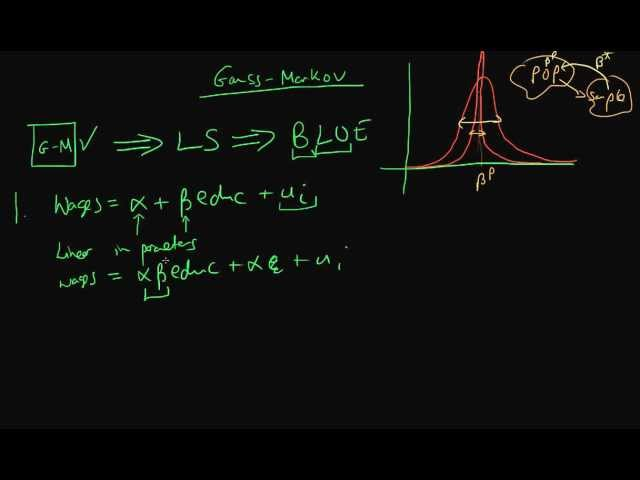﻿gauss markov assumptions part 1

## gauss markov assumptions part 1

this video details the first half of the gauss-markov assumptions, which are necessary for ols estimators to be blue.

i, in this video i am going to be talking about the gauss-markov assumptions in econometrics, and what their significance is. so, the gauss-markov assumptions are a set of criteria which were first created by the mathematicians carl-friedrich gauss, and andrei markov, which if they are upheld, then that says something about our ability to use least-squared estimators on the sample data. well, it says that those least-squares estimators are in fact blue. but, what does it mean for an estimator to be blue? well, it means that there are no other linear unbiased estimators which have a lower sampling variance than that particular estimator. so, i can illustrate this graphically, imagine i have a sampling distribution of one estimator which looks like that. and then i have another one which has the same sort of centre as the first one, except that it is slightly steeper towards the centre of the distribution. so, assuming that both of these are unbiased, so they are both centered around the true population parameter, we can see that the second estimator has a lower sampling variance that the first. well, that means that, more often than not when i use my least-squared estimators - when i apply my least-squared estimators to the sample data, they are going to more often than not provide estimates of the true population parameter 'beta p', which are closer to 'beta p' than i would have got by using the first type of estimator. so, that's the significance in econometrics of the gauss-markov assumptions. but, what are the gauss-markov assumptions? there's no particular order to the gauss-markov assumptions, but i am going to label them here so that means that i can refer to them in the future. the first gauss-markov assumption has to do with the population process, so assuming that there is some population process which connects wages with the number of years of education, although education doesn't exactly determine wages, because there's some sort of error term here. this is an example of a model which is linear in parameters, so that means that it's linear in alpha and beta. so this is the first gauss-markov assumption which says that our population process has to be linear in parameters. note that if i had this type of model where i had wages equal to alpha times beta times the number of years of education plus alpha...well just alpha on its own - this would be nonlinear in parameters because this implies some sort of multiplicative effect between alpha and beta. or if i had beta-squared here, that would also be nonlinear in parameters. note that however that being linear in parameters does not mean that i cannot have a variable in our model which is nonlinear. so, actually just having education squared in our model, rather than just education, that is absolutely fine under the assumption of 'linearity in parameters'. it just means that i am not allowed to have a model which has nonlinear parameters within it. so, that's the first gauss-markov condition - the second condition is that we have a set of sample data - x and y which are a random sample from the population. so what does that actually mean? well, it means that within our population, a random sample occurs if each individual within our population is equally likely to be picked, when i take the sample. that's what we mean by a random sample. but, it also implicitly means that not only are each person in the population equally likely to be picked, but it means that all of our points come from the same population. so they come from the same population process which in this context might be wages being equal to alpha plus beta times education. plus some error. the third condition is perhaps the most important of the gauss-markov conditions, which is the zero conditional mean of errors. so what does this actually mean? well mathematically it means that the expectation of our error term in our population given our x term, which in this case is education has got to be equal to zero. well, what does this mean practically? well it means that if i know someone's level of education that does not help me to predict whether they will be above or below the average population regression line. so that's what it means for there to be a zero conditional mean of error. and this is perhaps the most important of the gauss-markov assumptions, for reasons which we'll come onto later. so, that concludes our first video looking into the gauss-markov assumptions. i'm going to, in the next video, explain the next three gauss-markov assumptions. check out https://ben-lambert.com/econometrics-course-problem-sets-and-data/ for course materials, and information regarding updates on each of the courses.